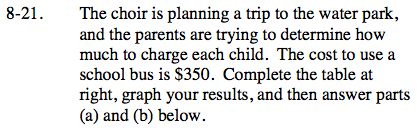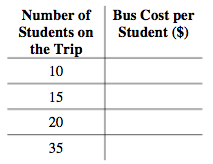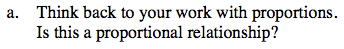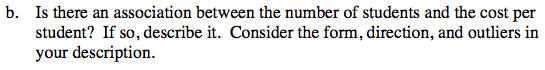Home > ACC7 > Chapter cc38 > Lesson cc38.1.2 > Problem8-21

8-21.
1.  Number of Students on the Trip Bus Cost Per Student ($) 10 15 20 35 The choir is planning a trip to the water park, and the parents are trying to determine how much to charge each child. The cost to use a school bus is$350. Complete the table at right, graph your results, and then answer parts (a) and (b) below. 8-21 HW eTool (Desmos). Homework Help ✎

1. Think back to your work with proportions. Is this a proportional relationship?

2. Is there an association between the number of students and the cost per student? If so, describe it. Consider the form, direction, and outliers in your description.To complete the table, divide 350 by the number of students.

Graphs of proportional relationships can pass through the point (0, 0). Does your graph look like it will pass through (0, 0)?For every student added, the trip costs less for everyone.

There is a non-linear negative association.

Use the eTool below to help complete the table and graph the problem.
Click the link at right for the full version of the eTool: CC3 8-21 HW eTool